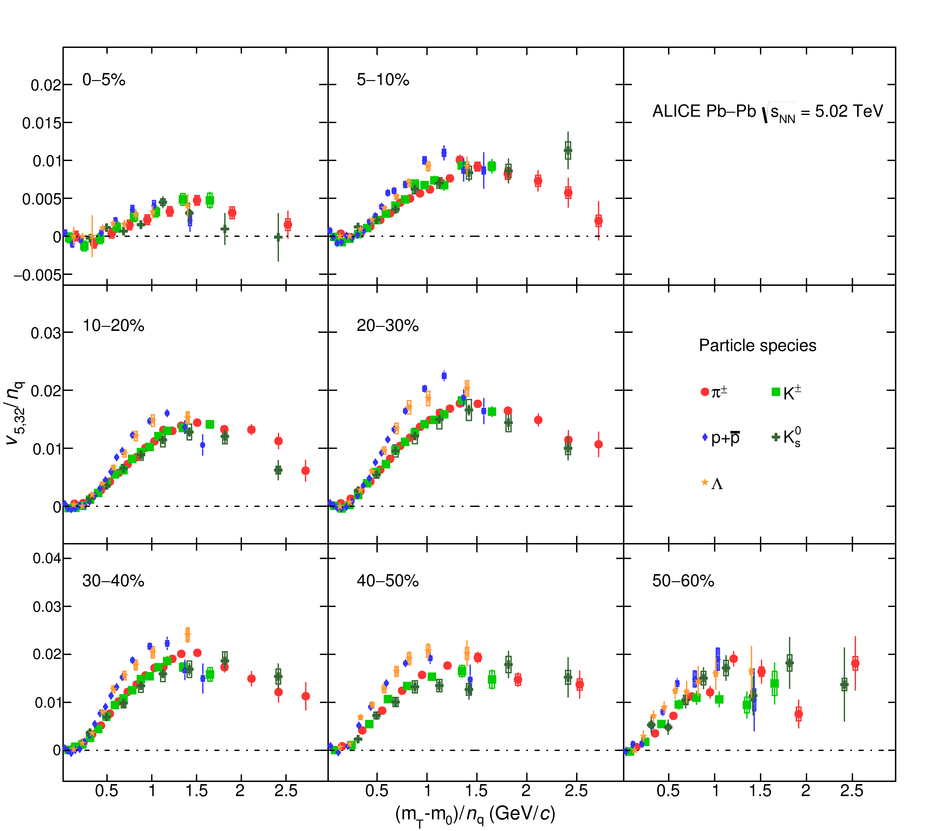# Figure 23

 The $(m_{\rm{T}} - m_{0})/n_{q}$-dependence of $v_{5,32}/n_{q}$ for different particle species grouped into different centrality intervals of Pb--Pb collisions \sNN. Statistical and systematic uncertainties are shown as bars and boxes, respectively. It is seen that the $\rm KE_{T}$ scaling holds for $v_{5,32}$ at an approximate level.